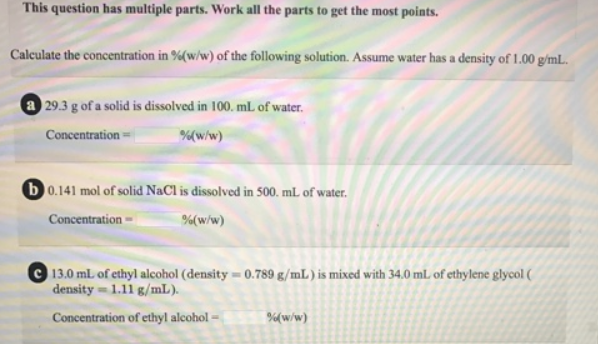# Problem: This question has multiple parts. Work all the parts to get the most points.Calculate the concentration in %(w/w) of the following solution. Assume water has a density of 1.00 g/mL. a. 29.3 g of a solid is dissolved in 100. mL of water. b. 0.141 mol of solid NaCl is dissolved in 500. mL of water. c. 13.0 mL of ethyl alcohol (density = 0.789 g/mL) is mixed with 34.0 mL of ethylene glycol (density = 1.11 g/mL).

###### FREE Expert Solution
91% (332 ratings)###### Problem Details

This question has multiple parts. Work all the parts to get the most points.

Calculate the concentration in %(w/w) of the following solution. Assume water has a density of 1.00 g/mL.

a. 29.3 g of a solid is dissolved in 100. mL of water.

b. 0.141 mol of solid NaCl is dissolved in 500. mL of water.

c. 13.0 mL of ethyl alcohol (density = 0.789 g/mL) is mixed with 34.0 mL of ethylene glycol (density = 1.11 g/mL).